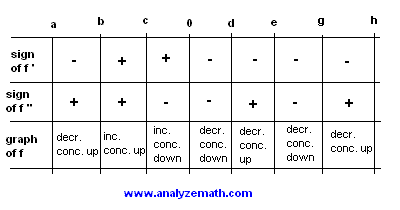# Questions on Concavity and Inflection Points

Questions with detailed answers on concavity and inflection point of graphs of functions.

### Question 1

What is the concavity of the graph of the general quadratic function given by
f(x) = a x 2 + b x + c

Solution to Question 1:

• We first calculate the first and second derivative of function f
f '(x) = 2 a x + b
f "(x) = 2 a
• The sign of f "(x) is the same as the sign of a. The graph of f which is called a parabola will be concave up if a is positive and concave down if a is negative.

### Question 2

Find all intervals on which function f given by
f(x) = sin x

is concave up.

Solution to Question 2:

• The first and second derivatives of function f are given by
f '(x) = cos x
f "(x) = - sin x
• We now study the sign of f "(x) by solving the inequality
- sin x > 0
• Multiply both sides by -1 to obtain
sin x < 0
• Let us study sin x < 0 over the interval [0, 2π] which represents one period of sin x. sin x is negative for x in the interval [π , 2 π]. Hence sin x is concave up on the interval (π , 2 π). We now extend this result over the domain of f which is (-∞ , +∞) and say that sin x is concave up on all intervals of the form (π + 2π k , 2π + 2π k) where k is an integer taking the values 0, -1, +1, -2, +2,...

### Question 3

The graph of f '(x) is shown below for x in the closed interval [a , g]. On which interval (s) is function f both decreasing and concave down? Find any points of inflection.Solution to Question 3:

• The sign of f '(x) determines the interval of increase / decrease of a function f and the sign of f "(x) determines the concavity. We shall divide the whole interval [a , g] into smaller intervals and use a table to study the sign of f ' and the sign of f ".
• The sign of f ' is given by the graphs: parts of the graph below the x axis correspond to f '(x) negative and parts above the x axis correspond to f '(x) positive. See table below.
• An interval over which f ' increases correspond to f "(x) positive and an interval over which f ' decreases correspond to f "(x) negative. See table below.• The table above shows that f is decreasing and concave down over the intervals (0 , d) and (e , g).
• The points of inflection occur when there is a change in concavity. Hence (c , f(c)) , (d , f(d)) , (e , f(e)) and (f , f(g)) are all points of inflection.

### Question 4

Determine all inflection points of function f defined by
f(x) = 4 x 4 - x 3 + 2

Solution to Question 4:

• In order to determine the points of inflection of function f, we need to calculate the second derivative f " and study its sign. This gives the concavity of the graph of f and therefore any points of inflection.
f '(x) = 16 x 3 - 3 x 2
f "(x) = 48 x 2 - 6 x
= 6x (8x - 1)
• The table below shows the signs of 6x and 8x - 1 and that of f " which is the product of 6x and 8x - 1. Also the concavity is shown. The points of inflection are located where there is a change in concavity. Hence the points
(0 , f(0)) = (0 , 2)
and (1/8 , f(1/8)) = (1/8 , 2047/1024)
are points of inflection.### Question 5

Determine the inflection point of
f(x) = - x 3 + 3 x 2 + 1

then use it to determine the inflection point of
g(x) = - (x - 2) 3 + 3 (x - 2) 2 + 1
.

Solution to Question 5:

• The first and second derivatives of f are given by
f '(x) = - 3 x 2 + 6 x
f "(x) = -6 x + 6
• Any change of sign of f " gives an inflection point. The sign of
f " changes at x = 1. Hence the inflection point of f is at
(1 , f(1)) = (1 , 3).

• A close examination of g(x) shows that g(x) = f(x - 2) which means that the graph of g is the graph of f shifted horizontally 2 units to the right. But if the graph of a function is shifted 2 units to the right all points, including any inflection point, on the graph of f are shifted 2 units to the right so that the inflection point of g is at (1+2 , 3) = (3 , 3).

## More References on Calculus

questions with answers and tutorials and problems .